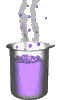Science class online
Chemistry and physics lessons

# ElectricityFree online electricity lessons for elementary school, middle school and high school. Take advantage of scientific resources that can be used freely to discover electricity, the science that explains electrical phenomenons and electric circuits
 Electrical components Two-terminal electronic components Basic electrical components Diodes What's a resistor ? How to determine a resistor value ? Resistors effects in circuits How to use a resistor ? Characteristic curve of a resistor How to build simple circuits and draw diagrams How to build a basic electrical circuit HThe electric current Conductors and insulators Direction of electric current in a circuit The dangers of electricity Current intensity - units how to measure current ? The Voltage The voltage and its units How to measure a voltage ? Voltage in open and closed circuits Rated current and voltage for a lamp Alternating voltage and current Alternating currents effects on led What are alternating current and voltage ? Periodic alternating voltage and its properties Oscilloscope Oscillogram Frequency Measuring RMS voltage with a voltmeter Serie circuits What's a serie circuit ? Series circuit properties Short circuit in  series circuit Current law in series circuits Voltage law in series circuits Parallel circuits What's a parallel circuit ? Some parallel circuits properties Short circuits in parallel circuits Nodes and branches in parallel circuits Current laws in parallel circuits Voltage laws in parallel circuit Laws of electricity Ohm's law Current laws in series circuits Voltage laws in series circuits Current laws in parallel circuits Voltage laws in parallel circuits Generating electricity Voltage for coil wires What is an alternator ? Alternating currents and voltages Generating electricity in power plants Electric power and energy Electric power and power rating Electric power received by an electrical device Electric power consumption by an electrical device Relationship between Electric power and energy